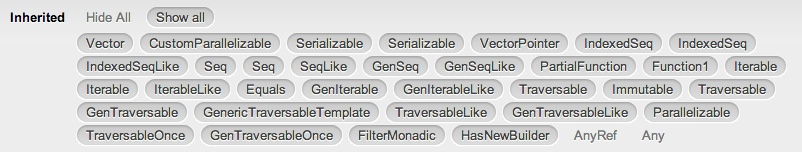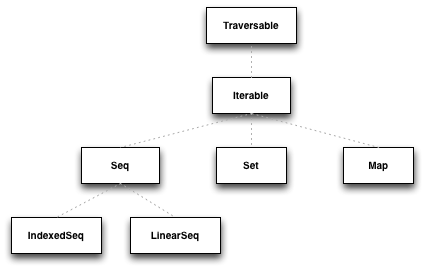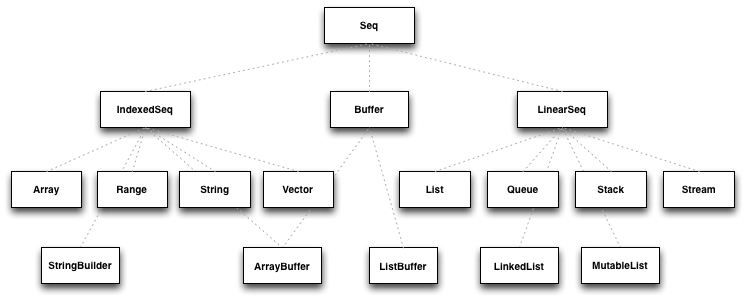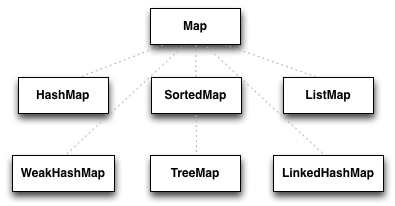# Understanding the Scala collections hierarchy

This is an excerpt from the Scala Cookbook. (This is Recipe 10.1.)

Overview: The Scala collections hierarchy is very rich (both deep and wide), and understanding how it’s organized can be helpful when choosing a collection to solve a problem.

## Solution

Figure 10-1, which shows the traits from which the `Vector` class inherits, demonstrates some of the complexity of the Scala collections hierarchy.Figure 10-1. The traits inherited by the `Vector` class

Because Scala classes can inherit from traits, and well-designed traits are granular, a class hierarchy can look like this. However, don’t let Figure 10-1 throw you for a loop: you don’t need to know all those traits to use a `Vector`. In fact, using a `Vector` is straightforward:

```val v = Vector(1, 2, 3)
v.sum                   // 6
v.filter(_ > 1)         // Vector(2, 3)
v.map(_ * 2)            // Vector(2, 4, 6)```

At a high level, Scala’s collection classes begin with the `Traversable` and `Iterable` traits, and extend into the three main categories of sequences (`Seq`), sets (`Set`), and maps (`Map`). Sequences further branch off into indexed and linear sequences, as shown in Figure 10-2.Figure 10-2. A high-level view of the Scala collections

The `Traversable` trait lets you traverse an entire collection, and its Scaladoc states that it “implements the behavior common to all collections in terms of a `foreach` method,” which lets you traverse the collection repeatedly.

The `Iterable` trait defines an iterator, which lets you loop through a collection’s elements one at a time, but when using an iterator, the collection can be traversed only once, because each element is consumed during the iteration process.

## Sequences

Digging a little deeper into the sequence hierarchy, Scala contains a large number of sequences, many of which are shown in Figure 10-3.Figure 10-3. A portion of the Scala sequence hierarchy

These traits and classes are described in Table 10-1 through Table 10-4 of the Scala Cookbook.

As shown in Figure 10-3, sequences branch off into two main categories: indexed sequences and linear sequences (linked lists). An `IndexedSeq` indicates that random access of elements is efficient, such as accessing an `Array` element as `arr(5000)`. By default, specifying that you want an `IndexedSeq` with Scala 2.10.x creates a `Vector`:

```scala> val x = IndexedSeq(1,2,3)
x: IndexedSeq[Int] = Vector(1, 2, 3)```

A `LinearSeq` implies that the collection can be efficiently split into head and tail components, and it’s common to work with them using the `head`, `tail`, and `isEmpty` methods. Note that creating a `LinearSeq` creates a `List`, which is a singly-linked list:

```scala> val seq = scala.collection.immutable.LinearSeq(1,2,3)
seq: scala.collection.immutable.LinearSeq[Int] = List(1, 2, 3)```

## Maps

Like a Java `Map`, Ruby `Hash`, or Python dictionary, a Scala `Map` is a collection of key/value pairs, where all the keys must be unique. The most common map classes are shown in Figure 10-4.Figure 10-4. Common Map classes

`Map` traits and classes are discussed in Table 10-5. When you just need a simple, immutable map, you can create one without requiring an import:

```scala> val m = Map(1 -> "a", 2 -> "b")
m: scala.collection.immutable.Map[Int,java.lang.String] = Map(1 -> a, 2 -> b)```

The mutable map is not in scope by default, so you must import it (or specify its full path) to use it:

```scala> val m = collection.mutable.Map(1 -> "a", 2 -> "b")
m: scala.collection.mutable.Map[Int,String] = Map(2 -> b, 1 -> a)```

## Sets

Like a Java `Set`, a Scala `Set` is a collection of unique elements. The common set classes are shown in Figure 10-5.Figure 10-5. Common Scala set classes

`Set` traits and classes are discussed in Table 10-6, but as a quick preview, if you just need an immutable set, you can create it like this, without needing an import statement:

```scala> val set = Set(1, 2, 3)
set: scala.collection.immutable.Set[Int] = Set(1, 2, 3)```

Just like a map, if you want to use a mutable set, you must import it, or specify its complete path:

```scala> val s = collection.mutable.Set(1, 2, 3)
s: scala.collection.mutable.Set[Int] = Set(1, 2, 3)```

## More collection classes

There are many additional collection traits and classes, including `Stream`, `Queue`, `Stack`, and `Range`. You can also create views on collections (like a database view); use iterators; and work with the `Option`, `Some`, and `None` types as collections. All of these classes (and objects) are demonstrated in this and the next chapter.

## Strict and lazy collections

Collections can also be thought of in terms of being strict or lazy. See Recipe 10.2 for a discussion of these terms.

## The Scala Cookbook

This tutorial is sponsored by the Scala Cookbook, which I wrote for O’Reilly:You can find the Scala Cookbook at these locations: